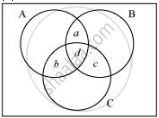Department of Pre-University Education, Karnataka course PUC Karnataka Science Class 11
Share

# In a Survey of 60 People, It Was Found that 25 People Read Newspaper H, 26 Read Newspaper T, 26 Read Newspaper I, 9 Read Both H and I, - Mathematics

ConceptPractical Problems on Union and Intersection of Two Sets

#### Question

In a survey of 60 people, it was found that 25 people read newspaper H, 26 read newspaper T, 26 read newspaper I, 9 read both H and I,11 read both H and T, 8 read both T and I, 3 read all three newspapers. Find:

(i) the number of people who read at least one of the newspapers.

(ii) the number of people who read exactly one newspaper.

#### Solution

Let A be the set of people who read newspaper H.

Let B be the set of people who read newspaper T.

Let C be the set of people who read newspaper I.

Accordingly, n(A) = 25, n(B) = 26, and n(C) = 26

n(A ∩ C) = 9, n(A ∩ B) = 11, and n(B ∩ C) = 8

n(A ∩ B ∩ C) = 3

Let U be the set of people who took part in the survey.

(i) Accordingly,

n(A ∪ B ∪ C) = n(A) + n(B) + n(C) – n(A ∩ B) – n(B ∩ C) – n(C ∩ A) + n(A ∩ B ∩ C)

= 25 + 26 + 26 – 11 – 8 – 9 + 3

= 52

Hence, 52 people read at least one of the newspapers.

(ii) Let a be the number of people who read newspapers H and T only.Let b denote the number of people who read newspapers I and H only.

Let c denote the number of people who read newspapers T and I only.

Let d denote the number of people who read all three newspapers.

Accordingly, d = n(A ∩ B ∩ C) = 3

Now, n(A ∩ B) = a + d

n(B ∩ C) = c + d

n(C ∩ A) = b + d

∴ a + d + c + d + b + d = 11 + 8 + 9 = 28

⇒ a + b + c + d = 28 – 2d = 28 – 6 = 22

Hence, (52 – 22) = 30 people read exactly one newspaper.

Is there an error in this question or solution?

#### Video TutorialsVIEW ALL 

Solution In a Survey of 60 People, It Was Found that 25 People Read Newspaper H, 26 Read Newspaper T, 26 Read Newspaper I, 9 Read Both H and I, Concept: Practical Problems on Union and Intersection of Two Sets.
S